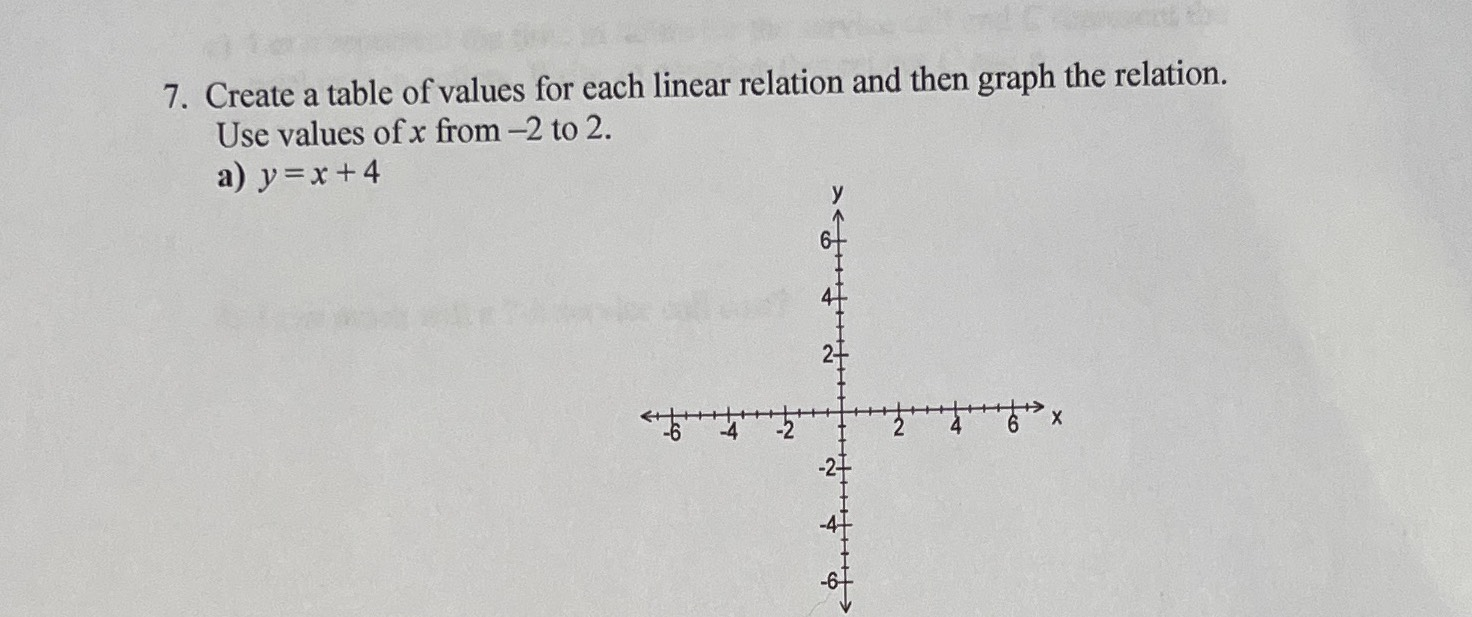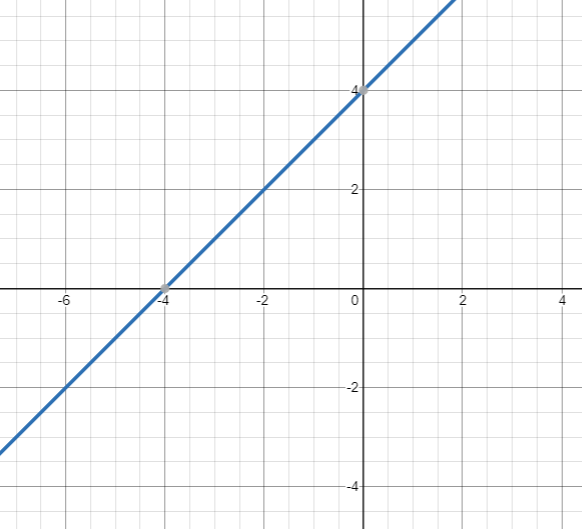### ¿Todavía tienes preguntas de matemáticas?

Pregunte a nuestros tutores expertos
Algebra
Pregunta7. Create a table of values for each linear relation and then graph the relation. Use values of $$x$$ from $$- 2$$ to $$2$$ . a) $$y = x + 4$$

$$(- 2,\ 2),(- 1,\ 3).(0,\ 4),(1,\ 5),(2,\ 6)$$